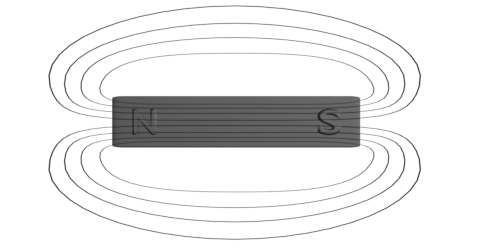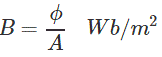# Magnetic Flux

We define magnetic flux as the total number of magnetic lines of force in a magnetic field.

If we place an imaginary isolated unit north pole in a magnetic field it will experience a repulsive force from north pole and an attractive force from south pole of the magnet which has created the field. Due to these both forces, acting on the isolated unit north pole, the north pole will move along a particular path in the field if the pole is free to do so. If we place the same isolated unit north pole at different distance from the magnet in the field, it may follow a different path of travelling.

We call these paths of travelling of the unit north pole in the field, as lines of force. As we can place this imaginary isolated unit north pole at infinite number of points in the field, there may be infinite numbers of lines of force in the field. But visualize a magnetic field with infinite number of lines of force is useless for any scientific calculation. So we have to develop some unique concept, so that we can represent a magnetic field according to its entire strength. We take the unit of magnetic flux as weber. If a field has φ weber flux, it means the field has total φ number of lines of force. Like isolated north pole, the concept of lines of force in a magnetic field is also imaginary. It does not has any physical existence. This is only used for different magnetic calculation and for explaining different magnetic properties.## Properties of Magnetic Flux

1. Magnetic flux of a filed is considered as the total number of magnetic lines of force in the field. These are also called magnetic flux lines.
2. Each magnetic flux line is closed loop.
3. Each magnetic flux line starts from north pole of a magnet and comes to the south pole through the field and continues from south pole to north pole in the body of the magnet.
4. No two flux lines cross each other.
5. Two similar lines of force travel side by side but repeal each other.
6. The lines of force are stretched like elastic cord.

## Magnetic Flux Density

The number of magnetic lines of force passing through a unit area surface perpendicular to the magnetic field is called magnetic flux density. If total φ Weber flux perpendicularly through a surface of area A m2, Magnetic flux density of the field would be,We generally represent magnetic flux density by capital letter B.

Want To Learn Faster? 🎓
Get electrical articles delivered to your inbox every week.
No credit card required—it’s 100% free.